Question

# Suppose the call money rate is 4.5 percent, and you pay a spread of 2.5 percent...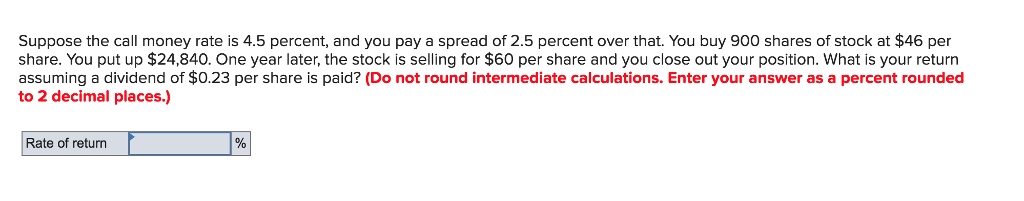Suppose the call money rate is 4.5 percent, and you pay a spread of 2.5 percent over that. You buy 900 shares of stock at \$46 per share. You put up \$24,840. One year later, the stock is selling for \$60 per share and you close out your position. What is your return assuming a dividend of \$0.23 per share is paid? (Do not round intermediate calculations. Enter your answer as a percent rounded to 2 decimal places.) Rate of return

 Initial investment \$ 24,840.00 Amount borrowed \$ 16,560.00 =900*46-24840 Interest expense \$    1,159.20 =16560*(4.5%+2.5%) Dollar return \$ 11,647.80 =900*(60+0.23-46)-1159.2 Rate of return 46.89% =11647.8/24840

#### Earn Coins

Coins can be redeemed for fabulous gifts.

Similar Homework Help Questions
• ### Suppose the call money rate is 6.8 percent, and you pay a spread of 1.9 percent...

Suppose the call money rate is 6.8 percent, and you pay a spread of 1.9 percent over that. You buy 1,200 shares at \$82 per share with an initial margin of 70 percent. One year later, the stock is selling for \$88 per share and you close out your position. What is your return assuming no dividends are paid? (Do not round intermediate calculations. Enter your answer as a percent rounded to 2 decimal places.) Rate of return %

• ### Suppose the call money rate is 6.8 percent, and you pay a spread of 1.9 percent...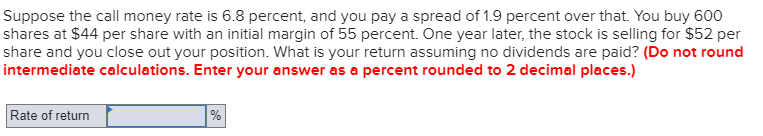Suppose the call money rate is 6.8 percent, and you pay a spread of 1.9 percent over that. You buy 600 shares at \$44 per share with an initial margin of 55 percent. One year later, the stock is selling for \$52 per share and you close out your position. What is your return assuming no dividends are paid? (Do not round intermediate calculations. Enter your answer as a percent rounded to 2 decimal places.) Rate of return

• ### Suppose the call money rate is 6.8 percent, and you pay a spread of 1.9 percent...

Suppose the call money rate is 6.8 percent, and you pay a spread of 1.9 percent over that. You buy 1,200 shares at \$82 per share with an initial margin of 70 percent. One year later, the stock is selling for \$88 per share and you close out your position. What is your return assuming no dividends are paid? Rate of return: _____

• ### Suppose the call money rate is 6.8 percent, and you pay a spread of 1.9 percent...

Suppose the call money rate is 6.8 percent, and you pay a spread of 1.9 percent over that. You buy 1000 shares at 63 dollars per share with an initial margin of 70 percent. One year later, the stock is selling for 68 per share and you close out your position. what is your return assuming no dividends are paid?

• ### Suppose the call money rate is 6.8 percent, and you pay a spread of 1.9 percent...

Suppose the call money rate is 6.8 percent, and you pay a spread of 1.9 percent over that. You buy 700 shares at \$89 per share with an initial margin of 70 percent. One year later, the stock is selling for \$97 per share and you close out your position. What is your return assuming no dividends are paid? (Do n

• ### Suppose the call money rate is 6.8 percent, and you pay a spread of 1.9 percent...

Suppose the call money rate is 6.8 percent, and you pay a spread of 1.9 percent over that. You buy 1,200 shares at \$82 per share with an initial margin of 70 percent. One year later, the stock is selling for \$88 per share and you close out your position. What is your return assuming no dividends are paid? Rate of return____ I tried 4.71% and it is not the answer

• ### Suppose the call money rate is 6.8 percent, and you pay a spread of 1.9 percent...

Suppose the call money rate is 6.8 percent, and you pay a spread of 1.9 percent over that. You buy 1,500 shares at \$50 per share with an initial margin of 25 percent. One year later, the stock is selling for \$58 per share, and you close out your position. What is your return assuming no dividends are paid? (Round your answer to 2 decimal places. )

• ### Check my work 15 Suppose the call money rate is 6.8 percent, and you pay a...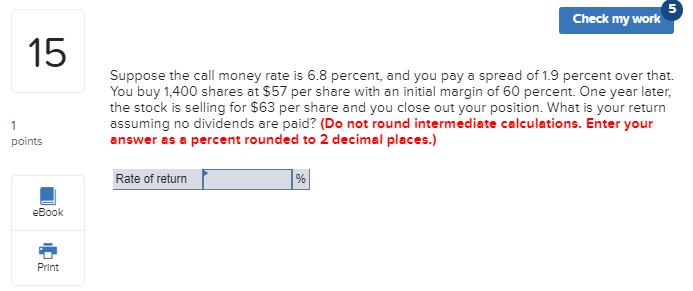Check my work 15 Suppose the call money rate is 6.8 percent, and you pay a spread of 1.9 percent over that. You buy 1,400 shares at \$57 per share with an initial margin of 60 percent. One year later, the stock is selling for \$63 per share and you close out your position. What is your return assuming no dividends are paid? (Do not round intermediate calculations. Enter your answer as a percent rounded to 2 decimal places.) points...

• ### please show your work :) 16. Suppose the call money rate is 6,8 percent, and you...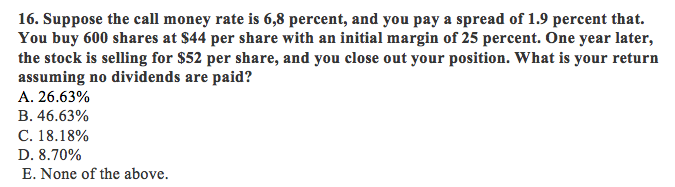please show your work :) 16. Suppose the call money rate is 6,8 percent, and you pay a spread of 1.9 percent that. You buy 600 shares at S44 per share with an initial margin of 25 percent. One year later, the stock is selling for \$52 per share, and you close out your position. What is your return assuming no dividends are paid? A. 26.63% B. 46.63% C. 18.18% D. 8.70% E. None of the above.

• ### can I have the answers for q15-16 112 If you want to keep your position one...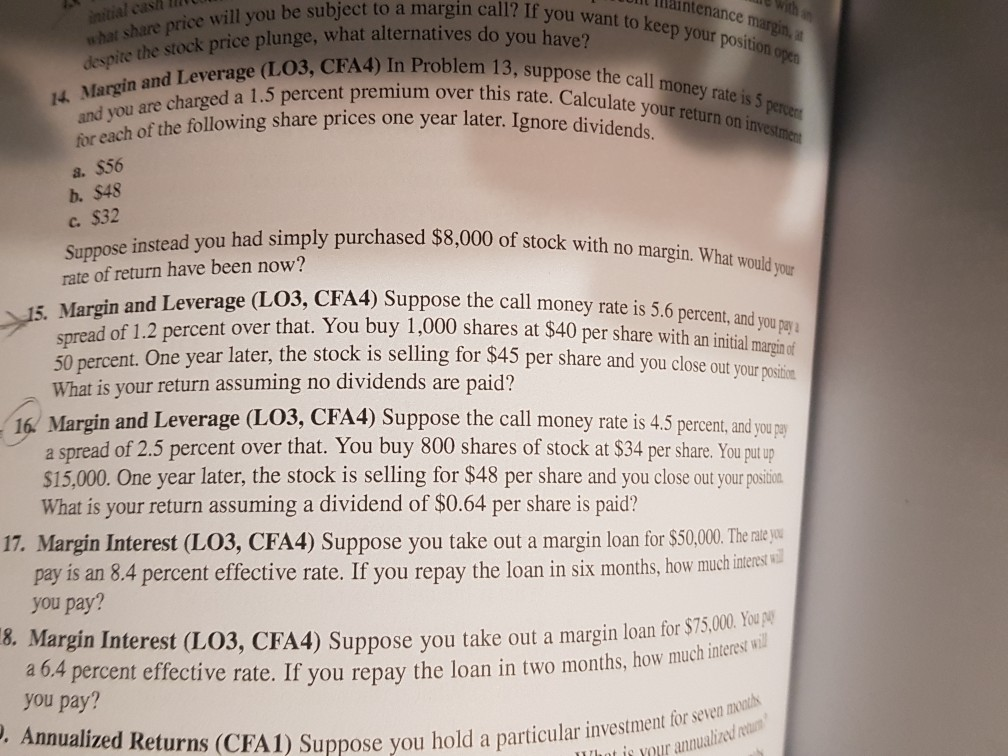can I have the answers for q15-16 112 If you want to keep your position one llaintenance margina Initial cash what share price will you b despite the stock price plunge, what alter 14 Margin and Leverage (LO3 and you are charged a 1.5 percen for each of the following share, a. 556 b. \$48 c. \$32 rice will you be subject to a margin call? If you olunge, what alternatives do you have? Leverage (LO3, CFA4) In Problem 13....